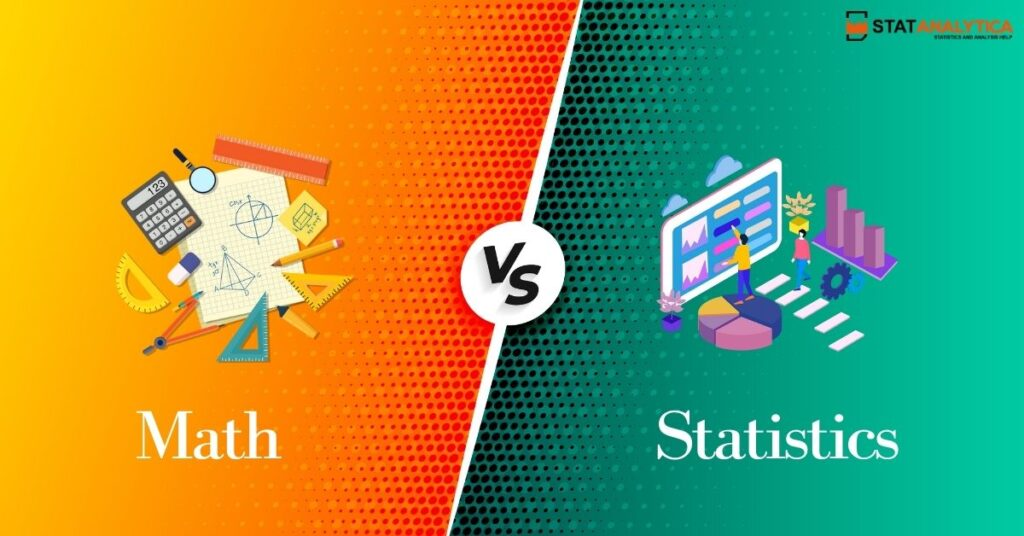# Math Vs Statistics: Top 9 Important Points One Should Know

For students who are confused about which to pursue in Mathematics vs Statistics, this blog will help you.

What is the difference between mathematics and statistics? or Math Vs Statistics is very common among students. Several students are not aware of the difference between mathematics and statistics. So, to understand the answer to this question, we have to go through the core concept of both.

Thus, in this blog, we will explore the concept of Math vs Statistics and know the difference between both.

Before digging into the comparison of math vs statistics. We will start discussing the definition of Math and Statistics.

## What exactly is mathematics?

The science and study of quality, structure, numbers, space, and change are known as mathematics. Mathematicians look for patterns, create new data, and show the truth by accurate answers from well-chosen assumptions and definitions.

There are numerous branches of mathematics in science connected to numbers, such as geometric shapes, algebra, and others. The name “mathematics” comes from Greek and means “tendencies to learn.”

## What exactly is Statistics?

Statistics is a department of applied mathematics. It collects, defines, analyzes, and judges conclusions from numerical data. Differential and integral calculus, linear algebra, and probability theory are used in statistics’ mathematical ideas.

There are two types of Statistics. Descriptive statistics, which explains the features of sample and population data. And inferential statistics, which uses those properties to test data and make conclusions.

## Interest Of Students in Math vs Statistics

Let’s see the below graph showing students’ interest in Mathematics and Statistics majors in the last 5 years.

As you see from the above graph, students’ interest in mathematics is more than in Statistics. But, in March 2020, it was noticed that the interest of students in Statistics was the highest in comparison to other years. But still, the trend of mathematics is higher than Statistics among students. It is the trend comparison between math vs statistics.

Now let’s move to discuss the difference between math vs Statistics.

## Math Vs Statistics

The following table shows the difference between both concepts.

## Career Differences for Math vs Statistics Major

While mathematicians and statisticians do a similar job. They applying mathematical and statistical approaches to data analysis. There are notable differences in their professional paths. For beginners, a statistician is a more extensive occupation in the United States than a mathematician. With 37,200 statistician employment vs 3,100 mathematician jobs.

Both fields are quickly developing. But statisticians are expected to grow faster and have a far bigger rise in overall job opportunities than mathematicians.

Mathematicians will likely have a 30 percent job growth over the next decade, resulting in 900 new employment chances. In contrast, statisticians will notice a 34 percent job growth rate, resulting in 12,600 new job chances.

Although there are fewer mathematicians than statisticians. Mathematicians make more money. Statisticians earn an average of \$84,060 over all industries, compared to the median pay of \$103,010 for mathematicians. It is the career comparison between Math vs Statistics.

Mathematicians earn a median annual income of \$120,840 in the highest-paying industries for these occupations, compared to \$103,630 for statisticians.

Now, what do you think!

## Is Statistics harder than Maths?

Let’s Discuss!

Discussing which one is harder entirely depends on the attitude, skills, and competence of the students.

Some students grow on thoughts and are horrified by any real-life applications. Mathematics makes sense to them.

Some students’ minds start to click only when they see a link between the problem and a real-life application or usage. And statistics make great use of credibility, which can be a complex subject to understand. Statistics may be a choice for students familiar with probability theory and making sense of data that has been measured, recorded, or quantified. You should know this while comparison math vs statistics.

## Conclusion

To conclude, I hope you understand the concept of Math Vs Statistics is clearly. Even though they appear to be somewhat similar on the surface, each has its own set of complexities. Both have multiple uses in daily life. To increase in each area or even in life, one must be very responsible for what one does. I can’t say how complicated a subject is because it depends on an individual’s IQ and practice. Choose your major between math vs statistics according to your strength and preference. Get the best math homework service from the experts at reasonable charges.